# help me with these problems and ill give you amazing ratings!! (must do all please) 11. Make a detailed graph of the function f)-1---on the interval |-4,1] by completing the following steps (a)...

help me with these problems and ill give you amazing ratings!! (must do all please)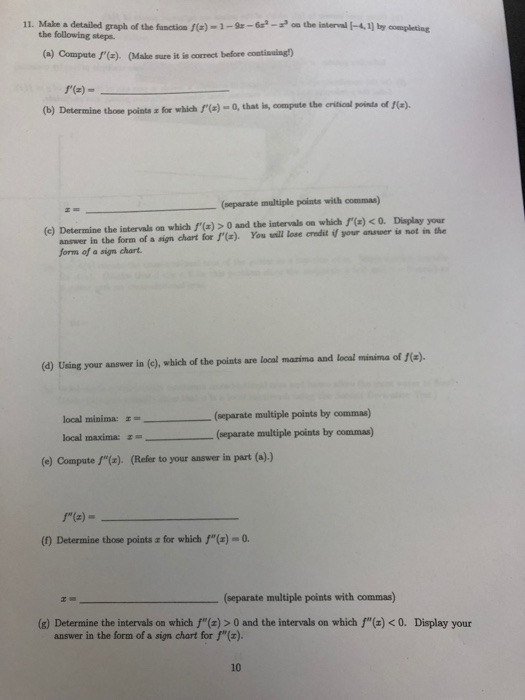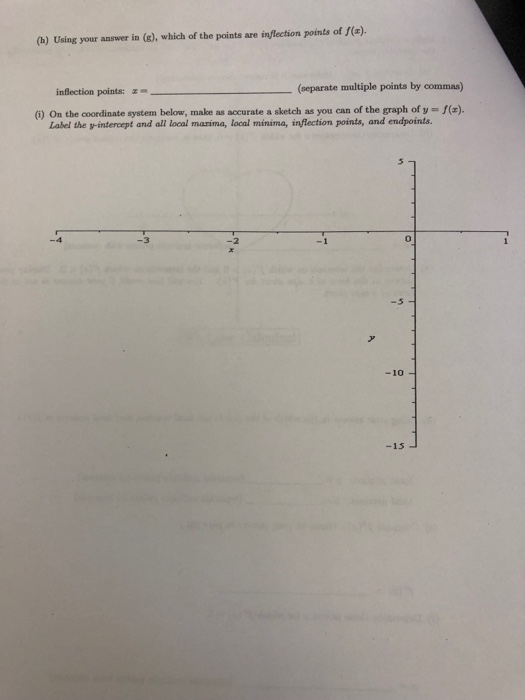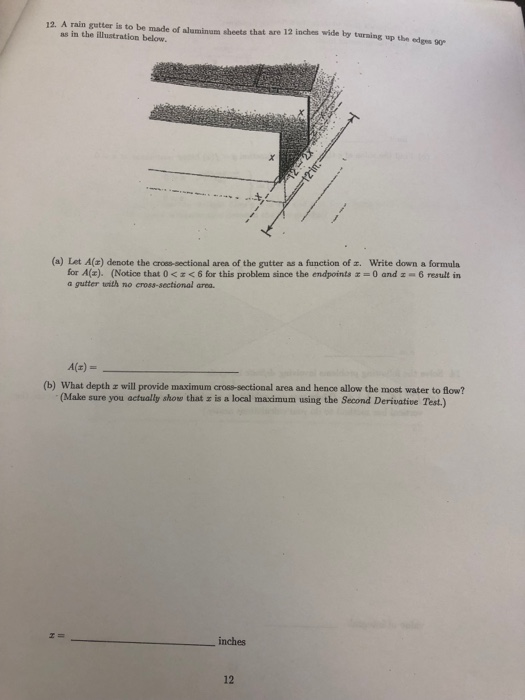11. Make a detailed graph of the function f)-1---on the interval |-4,1] by completing the following steps (a) Compate f(x). (Make sure it is correct before contisuing) (b) Determine those points a Determine those points x for which f(e)-o, that is, compate the eritical pointa of (oeparate multiple points with commas) answer in the form of a sign chart for ) You will lose credit if your anaseer is not in the form of a sign chart. (c) Determine the iatervals on which f(a)> 0 and the intervals on which f(a)0. Display your (d) Using your answer in (e), which of the points are local mazima and local minima of f(x). (separate multiple points by commas) (separate multiple points by commas) local minima: local maxima:z- (e) Compute /"(e). (Reler to your answer in part (o)) a) (1) Determine those points a for which f"(x) comma) (separate multiple points with エーーーーー ーーーーーーーー (g) Determine the intervals on which f"(z) > 0 and the intervals on which f"(z)b)c)-4

d)##### Add Answer of: help me with these problems and ill give you amazing ratings!! (must do all please) 11. Make a detailed graph of the function f)-1---on the interval |-4,1] by completing the following steps (a)...
Similar Homework Help Questions
• ### (a) Determine the intervals on which (x)> 0 and the intervals on which "(x) < o. Display your answer in the form of a sign chart for f"() (h) Using your answer in (8), which o...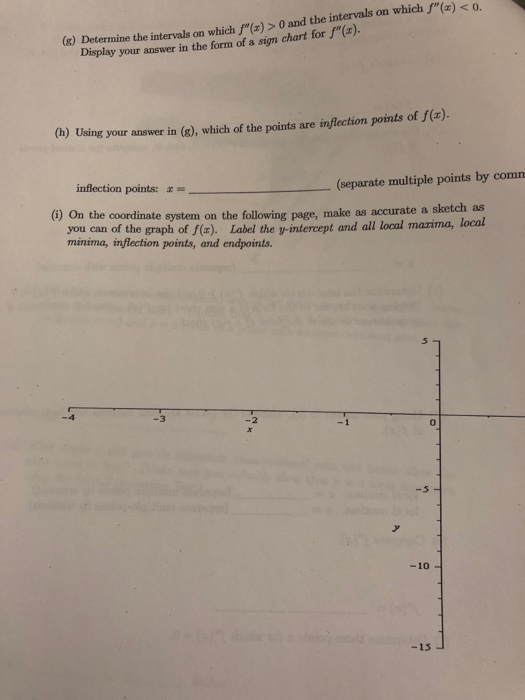(a) Determine the intervals on which (x)> 0 and the intervals on which "(x) < o. Display your answer in the form of a sign chart for f"() (h) Using your answer in (8), which of the points are inflection points of J(a). inflection points: (separate multiple points by comm (i) On the coordinate system on the following page, make as accurate a sketch as you can of the graph of f(x). Label the v-intercept and all local marima, minima,...

• ### help me with these problems and ill give you amazing ratings!! (must do all please) 7. Answer the following questions about continuity. (a) Write down the values of z at which the following func...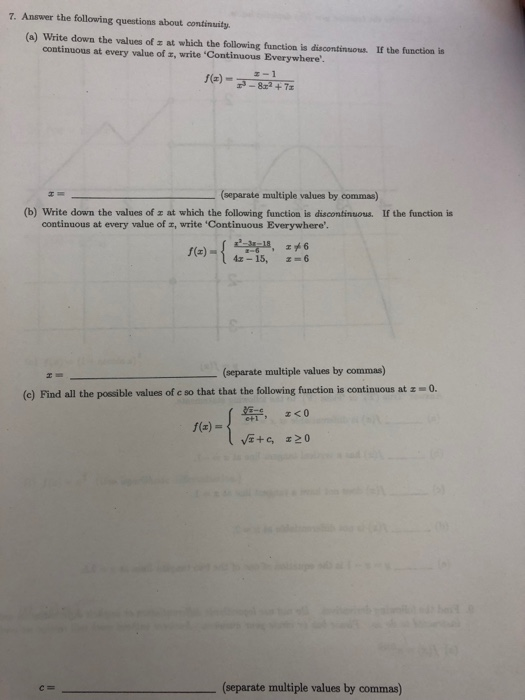help me with these problems and ill give you amazing ratings!! (must do all please) 7. Answer the following questions about continuity. (a) Write down the values of z at which the following function is discontinuous. If the function is continuous at every value of z, write 'Continuous Everywhere' f(a)1 (separate multiple values by commas) (b) Write down the values of z at which the following function is discontinuous. If the function is continuous at every value of z, write...

• ### Can I get some help with these few problems. Sorry for puttin all these questions in one post. I...

2.Suppose that f (x) =(7x - 6 )/( x + 2):(A) Use interval notation to indicate where f (x) is defined.If it is defined on more than one interval, enter the union of allintervals where f (x) is defined.Domain:(B) Find all intercepts. If there are no intercepts, enter None .If there are more than one, enter them separated by commas.x-intercept(s) =y-intercept(s) =(C) Find all critical values of f . If there are no critical values,enter None . If there are...

• ### Chapter 4 tch the graph. Each part Use the function below on the interval specified to answer the...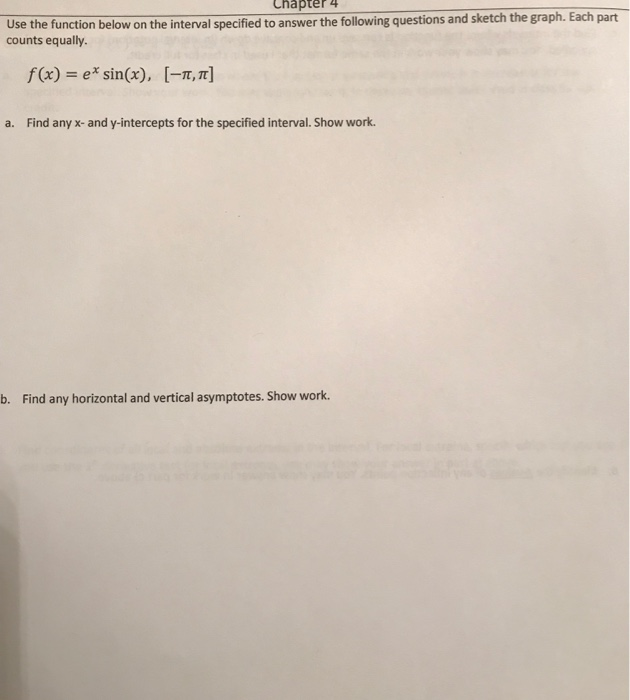Chapter 4 tch the graph. Each part Use the function below on the interval specified to answer the following questions and ske counts equally. f(x)= ex sin(x), [-π, π] a. Find any x- and y-intercepts for the specified interval. Show work. b. Find any horizontal and vertical asymptotes. Show work. c Give the intervals in interval notation where the function is increasing and where it is decreasing for the specified interval. Show your work. You may show your work in...

• ### Can someone help me with these functions max,min, inc,dec,etc. Thank You!!!

Suppose that f (x) =(x-4)/x^2(A) Use interval notation to indicate where f (x) is defined.If it is defined on more than one interval, enter the union of allintervals where f (x) is defined.Domain:(B) Find all intercepts. If there are no intercepts, enter None .x-intercept(s) =y-intercept(s) =(C) Find all critical values of f . If there are no critical values,enter None .Critical value(s) =(D) Use interval notation to indicate where f (x) is increasingor decreasing.Increasing:Decreasing:(E) Find the x-coordinates of all local...

• ### 1. You must SHOW ALL YOUR WORK on the test to receive full credit. 2. Print...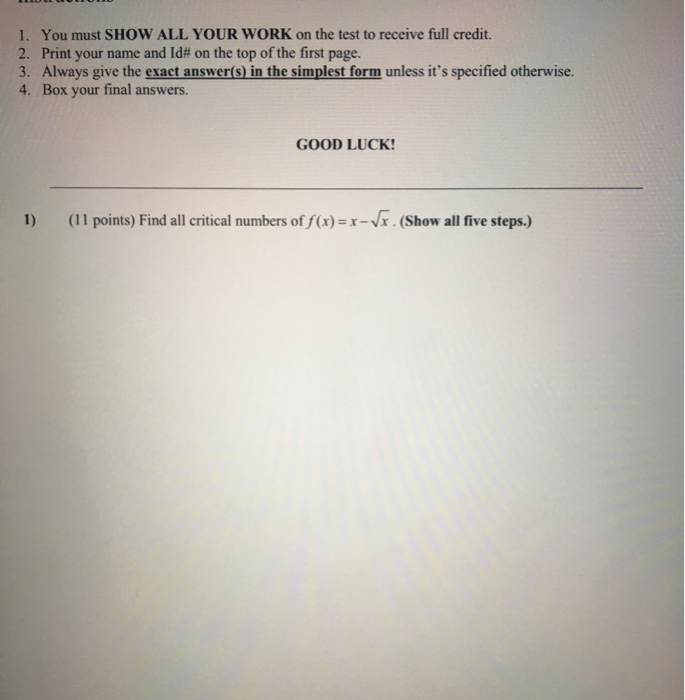1. You must SHOW ALL YOUR WORK on the test to receive full credit. 2. Print your name and Id# on the top of the first page. 3. Always give the exact answer(s) in the simplest form unless it's specified otherwise. 4. Box your final answers. GOOD LUCK! 1) (11 points) Find all critical numbers of f(x)=x-Vx. (Show all five steps.) 2) (11 points) The below figure shows the graph of derivative function f '(x) of a function f(x). The...

• ### Please show all work. Thank you! 2. Sections 4.3,4.5,4.6 Graphing:Consider the function f (x) = sin(2x)...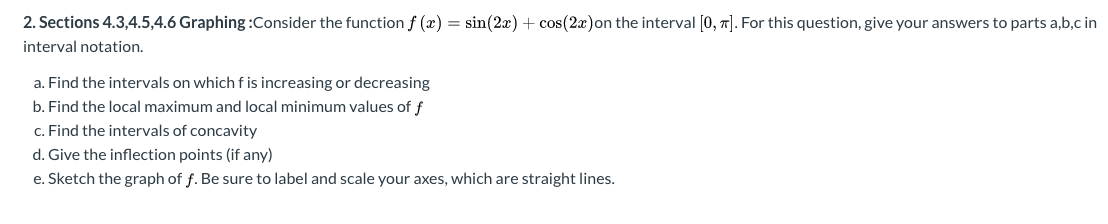Please show all work. Thank you! 2. Sections 4.3,4.5,4.6 Graphing:Consider the function f (x) = sin(2x) + cos(2x)on the interval [0, 1]. For this question, give your answers to parts a,b,c in interval notation. a. Find the intervals on which f is increasing or decreasing b. Find the local maximum and local minimum values of f c. Find the intervals of concavity d. Give the inflection points (if any) e. Sketch the graph of f. Be sure to label and...

• ### . Graphing Strategy Step 1 Analyze f() (i) Find the domain of f. (i) Find the intercepts. (i) Fin...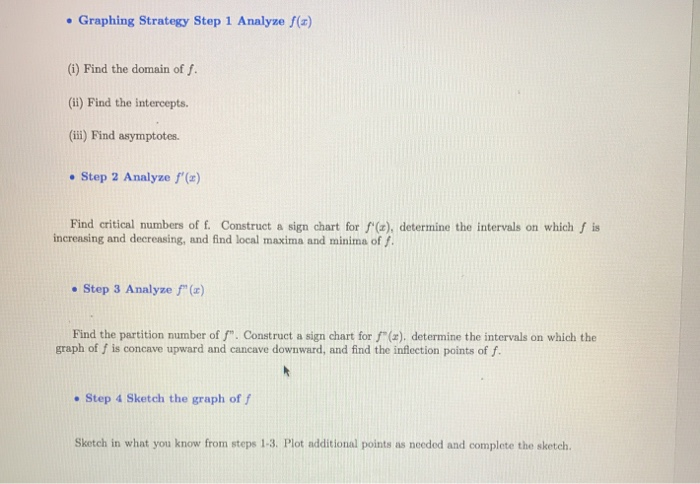. Graphing Strategy Step 1 Analyze f() (i) Find the domain of f. (i) Find the intercepts. (i) Find asymptotes . Step 2 Analyze f() Find critical numbers of f. Construct a sign chart for f(z), determine the intervals on which f is increasing and decreasing, and find local maxima and minima of ? . Step 3 Analyze f () Find the partition number of f. Construct a sign chart for f"(a),. determine the intervals on which the graph of...

• ### Let f() = 1+1 (a) Identify the domain off Instructions: Fill in the blank separating values...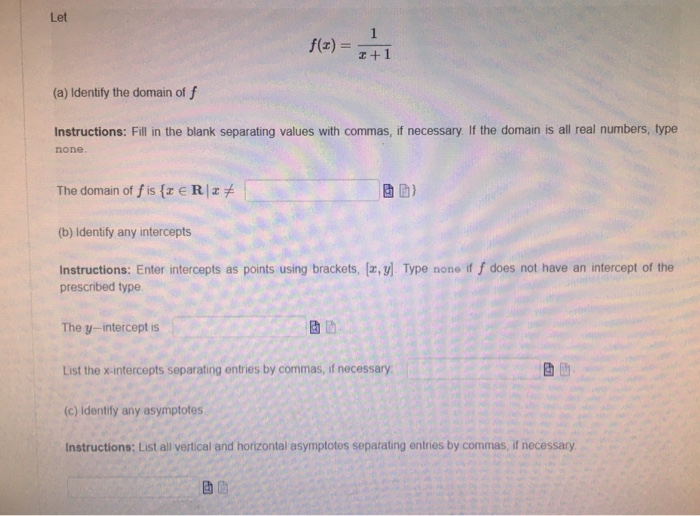Let f() = 1+1 (a) Identify the domain off Instructions: Fill in the blank separating values with commas, if necessary. If the domain is all real numbers, type none The domain of fis {x ERT (b) Identify any intercepts Instructions: Enter intercepts as points using brackets, [u, y). Type none if f does not have an intercept of the prescribed type The y-interceptis 3 List the x-intercepts separating entries by commas, if necessary (c) Identify any asymptotes Instructions: List all...

• ### can i het help with this question please The graph and equation of the function f are given. a. Use the graph to find any values at which f has a relative maximum, and use the equation to calcula...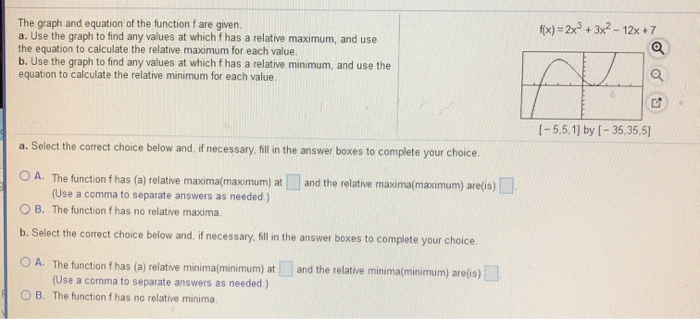can i het help with this question please The graph and equation of the function f are given. a. Use the graph to find any values at which f has a relative maximum, and use the equation to calculate the relative maximum for each value b. Use the graph to find any values at which f has a relative minimum, and use the equation to calculate the relative minimum for each value. fx)-2x3+3x2 -12x+7 I-5.5.1] by I-35,35,5] a. Select the...

Free Homework App